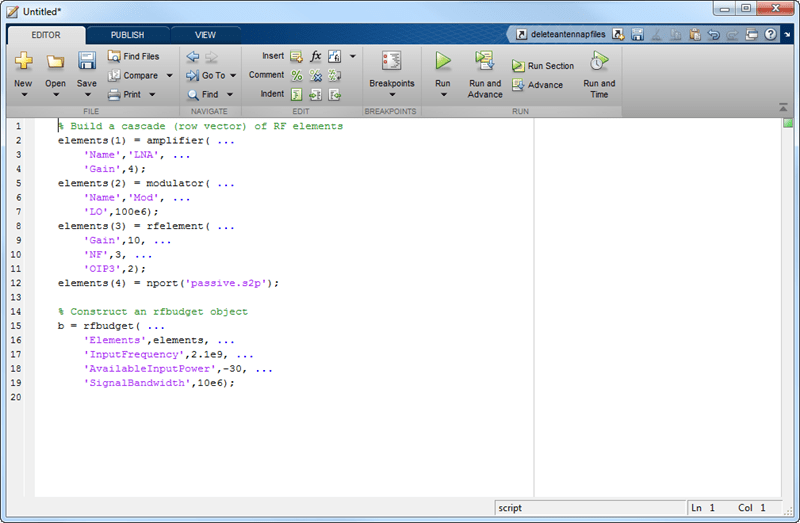exportScript

Export MATLAB code that generates RF budget object

Syntax

exportScript(rfobj)

Description

exportScript(rfobj) exports the MATLAB command-line code that generates an RF budget object. The script opens in an Untitled* window in the MATLAB editor.

Input Arguments

expand all

RF budget analysis object, specified as a object handle.

Examples

expand all

Create an RF budget object.

a = amplifier('Name','LNA','Gain',4);
m = modulator('ConverterType','Up','LO',100e6,'Name','Mod');
r = rfelement('Gain',10,'NF',3,'OIP3',2);
n = nport('passive.s2p');

Calculate the RF budget analysis.

b = rfbudget([a m r n],2.1e9,-30,10e6);

Export the analysis to a MATLAB script.

exportScript(b)